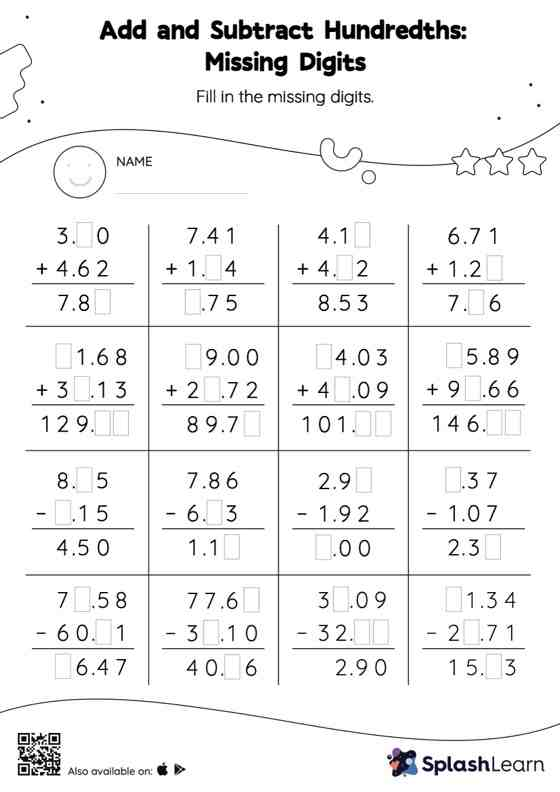# Add and Subtract Hundredths: Missing Digits Worksheet

Home > Add and Subtract Hundredths: Missing DigitsThis add and subtract hundredths worksheet consists of tasks to help your young mathematician develop fluency with addition and subtraction. In each problem, the numbers are laid out in the vertical format. In this format, pair of digits in each successive place are tackled one by one. This helps students follow a structured approach.